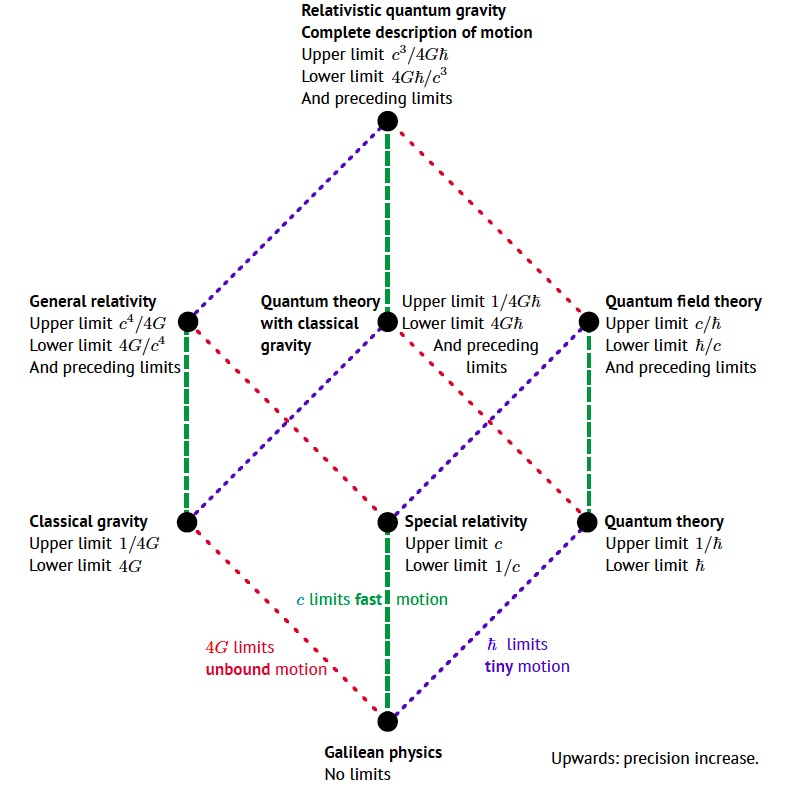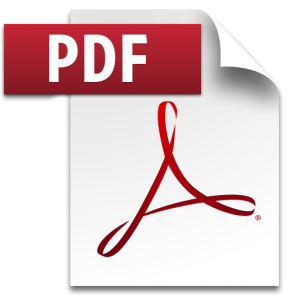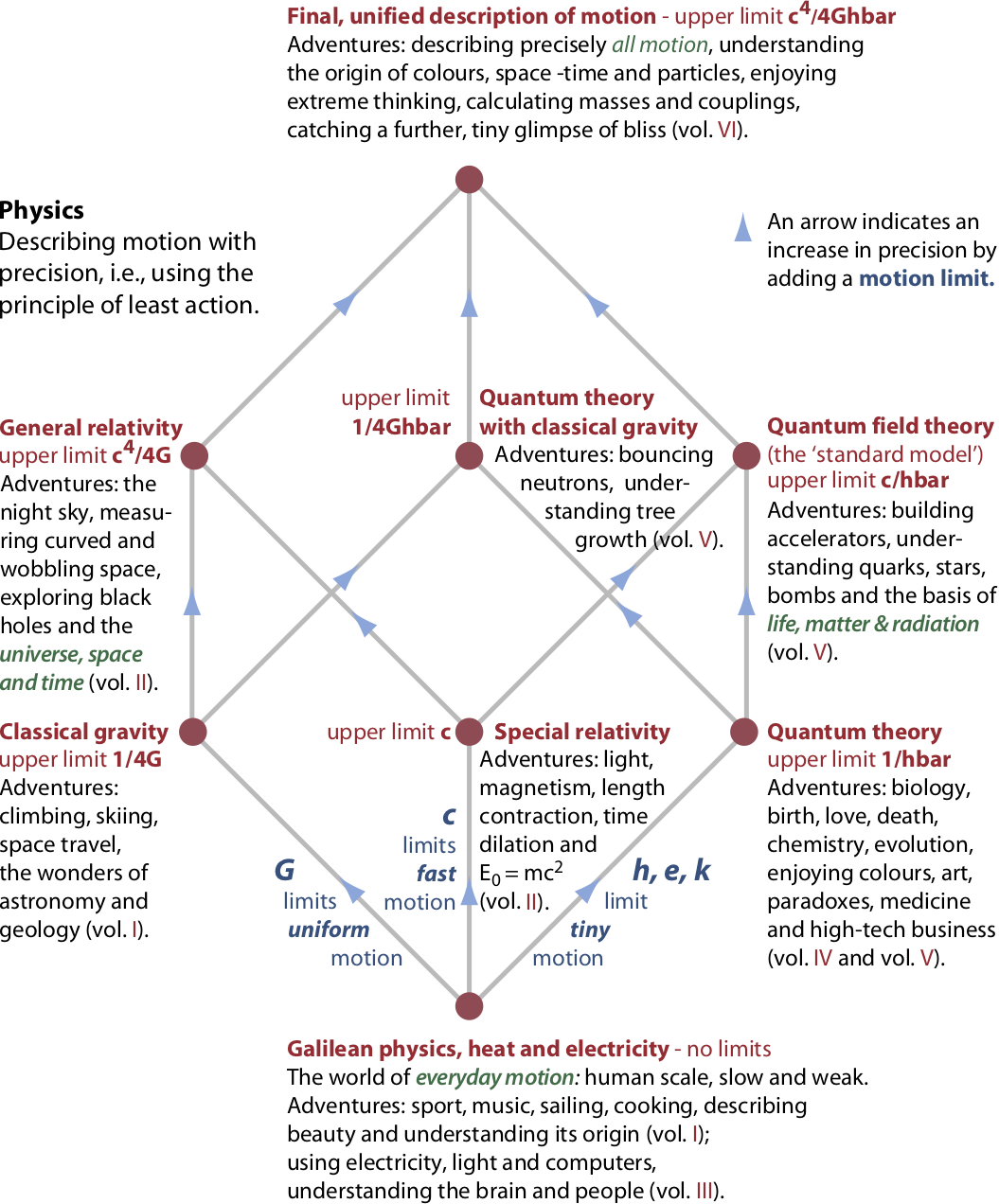### The Bronshtein limit cube of physicsThe main theories of physics can be distinguished by the combination of the speed of light c, the quantum of action ℏ and the gravitational constant G that they contain as a limit. Each limit value defines the respective theory. (Note that the limits are not unique; for example, both c and c2 are defining limits of special relativity.)

Physics starts at the bottom, where no limits are assumed, and proceeds towards the top, increasing precision at every corner. The topmost corner, the theory of relativistic quantum gravity, limits all observables. It provides the complete description of motion; and the description provides complete precision.

Several of the limits found in the cube are not well known. About the maximum force c4/4G, see the page and papers dedicated to the topic. About the "limit" 4G that distinguishes unbound and bound motion, see this preprint.The physics cube is often called Bronshtein cube (that is how he wrote his name himself) or also Bronstein cube. His cube dates from 1933.

In short, the Bronshtein cube with limits illustrates how the fields of physics are defined. It also shows that physics laws are simple.

*

### Consequences and predictions

Describing nature with limits implies that there are no infinite quantities in nature, neither infinitely large nor infinitely small.

The speed limit, its simplicity and its confirmation by experiment imply the prediction that there is no physics beyond special relativity.

The action limit, its simplicity and its confirmation by experiment imply the prediction that there is no physics beyond quantum theory.

The force limit, its simplicity and its confirmation by experiment imply the prediction that there is no physics beyond general relativity – thus no higher-order terms in the Lagrangian. Maximum force also suggests that the hoop conjecture is valid; both concepts are closely tied to horizons.

Combining the limits implies that the double Planck length is the shortest measurable length in nature. It does not mean that length values are multiples of the shortest length. Length is not quantized. Any length longer than the shortest one is possible in nature.

Together, all the limits also imply, as explained on a separate page, that all of physics can be summarized in 9 lines.

Again, the limits are not unique; other choices are also possible, using other powers of c, 4G and ℏ. For example, there is a maximum power c5/4G or a maximum mass flow rate c3/4G in general relativity, and a minimum length, a minimum area, a minimum volume, a maximum acceleration, or a maximum mass density in relativistic quantum gravity, the uppermost theory in the Bronshtein cube.

The Bronshtein cube is three-dimensional. (If the Boltzmann constant k is taken as separate line, one can extend it to a four-dimensional hypercube.) Understanding the origin of the number of dimensions of the physics cube is not straightforward, but most probably a red herring.

Together, the limits imply that the Planck scale is the central scale in nature, because they predict the lack of any trans-Planckian effects. In particular, the limits imply:

• There are no points in space or time,
• Space-time is effectively continuous, but at a fundamental scale,
• There is a smallest length,
• There are no higher dimensions,
• There are no lower dimensions,
• There is no space-time foam,
• There is no discrete space-time,
• There is no non-commutative space-time,
• There is no space-time lattice,
• There is no time-independent discrete space-time structure,
• There is no doubly special relativity,
• The equations of nature follow from inequalities involving limits.

In short, the Bronshtein cube imposes strict requirements on the complete description of motion. However, almost no present conjecture on the complete description of motion found in the research literature satisfies these requirements.

*

### Summary: What the Bronshtein cube shows and what it doesn't

Independently of whether one explores the Bronshtein cube or the Bronshtein hypercube – which includes the Boltzmann constant k or some other equivalent thermodynamic limit – one finds two conclusions.

1. The Bronshtein cube shows that the complete description of motion is near. The defining limits are known. In other words, fundamental physics is close to its completion.

2. However, the Bronshtein cube does not explain the origin of the elementary particles and their properties and the origin of the interactions and their properties. In particular, the origin of the values for particle masses, particle mixing angles, and the coupling constants remains open.

*

### Appendix: Another version of the Bronshtein cube

The physics cube also structures the adventure presented in the volumes of the free Motion Mountain Physics Textbook. This version has more details and mentions the adventures encountered in the different theories:*

### Bibliography

A longer writeup of this page is C. Schiller, The quadruple gravitational constant, the Bronshtein cube of limits, and implications. Download the 5-page preprint here.The history of the cube is confused. It also depends on the point of view taken, in particular on whether is it seen as a cube of theories, as a cube of fundamental constants, or, as is done here, as a cube of limits.

This often-cited reference is about c, G and h, but does not define the cube: G. Gamov, D. Ivanenko and L. Landau, Zh. Russ. Fiz. Khim. Obstva. Chast Fiz. 60 (1928) 13, (in Russian). Reprinted in G. Gamow, D. Ivanenko & L. Landau, World constants and limiting transition, Physics of Atomic Nuclei volume 65 (2002) 1373–1375. https://doi.org/10.1134/1.1495650

This often-cited reference is hard to find: M. Bronshtein, K voprosu o vozmozhnoy teorii mira kak tselogo [On a possible theory of the world as a whole], in Osnovnye problemy kosmicheskoy fiziki [Basic problems of cosmic physics], Kiev, ONTI (1934) 186-218 (in Russian).

This often-cited reference has nothing on the cube itself: M. Bronshtein, Physikalische Zeitschrift der Sowjetunion, 9, 140 (1936). https://doi.org/10.1007/s10714-011-1285-4

The papers by Okun and by Oriti are cited in the preprint.

*    *    *